# Least Squares Minimization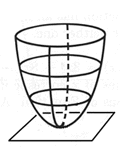The Linear Least Squares Minimization Problem

When we conduct an experiment we usually end up with measured data from which we would like to extract some information. Frequently the task is to find whether a particular model fits the data, or what combination of model data does describe the experimental data set best. This may be possible in a single minimization step or may require several cycles of successive refinement (as often the case in crystallography).

Example of a CD Spectra Fit

Let us discuss the simple case of a CD spectra fit. In a first approximation, a CD spectrum of a protein or polypeptide can be treated as a sum of three components: a-helical, b-sheet, and random coil contributions to the spectrum. At each wavelegth, the ellipticity (theta) of the spectrum will contain a linear combination of these components: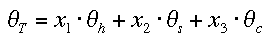(1)

where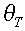is the total measured susceptibility,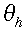the contribution from helix, s for sheet, c for coil, and the corresponding x the fraction of this contribution.

As we have three unknowns in this equation, a measurement at 3 points (different wavelengths) would suffice to solve the problem for x, the fraction of each contribution to the total measured signal. However, due to experimental error and imperfect model data the choice of points can mightily influence the result (a common source of subtle abuse in data interpretation). We usually have many more data points available from our measurement (e.g., a whole CD spectrum, sampled at 1 nm intervals from 190 to 250 nm). In this case, we can try to minimize the total deviation between all data points and calculated model values. This is done by a minimization of the sum of residuals squared (s.r.s.) which looks as follows in our case :(2).

A function has an extremum where its derivative is zero, i.e. in the one-dimensional case where the tangent to the graph is horizontal (zero slope, dx/dy = 0). The little icon on top shows a two-dimensional example, with a horizontal tangential plane defining the minimum for f(x,y). A minimum of our error function (i.e. the least error) can be found by setting the 3 partial derivatives to zero :(3).

Equation (2) is easy to derivatize by following the chain rule (or you can multipy eqn.3 out, or factor it and use the product rule). The following shows the derivation for x1(4)

With equation (4) and its analogues for x2 and x3, we have now 3 equations in 3 unknowns, which can be solved. We re-write the derivatives as normal equations (given here for x1 only)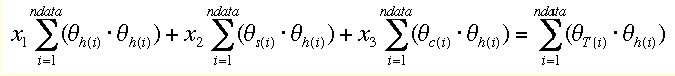which set up the matrix A of the normal equiations with its elementsetc. and vector b with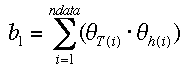etc.

We can solve the resulting general simultaneous equation problem(8)

easily by a standard method such as Gauss-Jordan elimination . The computer routine I selected returns the solution vector in b (i.e., the return values of b are the minimized values for x1, x2, and x3) and the inverse of A = A' in place of A. A' is also known as the correlation matrix C. The diagonal elements C(i,i) are the variance (squared standard deviation) of the fitting variables x, and the off-diagonal elements the correlation coefficients between them. However, when no weights are applied (in our example we do not know the standard deviation of the individual measurement), this value is not really meaningful. Sometimes eqn.(8) is written aswhere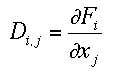which of course is equivalent (check!).

In some instances one might want to impose restraints and constraints on the problem. For example, nothing prevents our method from returning a negative value for one of the xes. This is mathematically perfectly ok, but does not make physical sense, there can't be a negative amount of, for example, helix in the sample. There are several texts that deal with these extensions to the LSQ method, a classic one is available from the ACA . A good description of the general LSQ problem, including the application of weights, can be found in . Of course, there is also the option to solve eqn.(8) by linear programming , which naturally imposes the constraint of positivity on the solutions x (set up one equation as target function and the other 2 as constraints).

In the implementation section we shall deal with the problem of negative fitting coefficients differently by using an iterative numerical method. As we do not have standard deviations for the data points, and thus no meaningful variance for our fitting parameters, we evaluate the r.m.s. deviation and an R-value as quality indicators for our fit.

 W.H.Press, S.A.Teukolsky, W.T.Vettering and B.P. Flannery, Numerical Recipes, 2nd edition, Cambridge University Press (1992)
 Least Squares Tutorial, ACA lecture note #1, American Crystallographic Association (1974)Back to Introduction
This World Wide Web site conceived and maintained by Bernhard Rupp
Last revised Dezember 27, 2009 01:40